# ML Aggarwal Class 6 Solutions for ICSE Maths Chapter 1 Knowing Our Numbers Ex 1.2

## ML Aggarwal Class 6 Solutions for ICSE Maths Chapter 1 Knowing Our Numbers Ex 1.2

Question 1.
Use the appropriate symbol < or > to fill in the blanks:
(i) 173 … 189
(ii) 1058 … 1074
(iii) 8315 … 8037
Solution: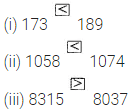Question 2.
In each of the following pairs of numbers, state which number is smaller:
(i) 553,503
(ii) 41338,1139
(iii) 25431,24531
Solution:Question 3.
Find the greatest and the smallest numbers in each row:
(i) 71834, 75284, 571, 2333, 594
(ii) 9853, 7691, 9999, 12002.
Solution:Question 4.
Arrange the following numbers in ascending order:
304, 340, 34, 43, 430
Solution: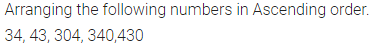Question 5.
Arrange the following numbers in descending order:
53, 7333, 553, 7529, 335.
Solution: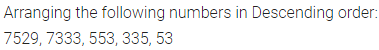Question 6.
Write all possible 2-digits numbers that can be formed by using the digits 2, 3 and 4. Repetition of digits is not allowed. Also find their sum.
Solution:Question 7.
Write all possible 3-digits numbers using the digits 3, 1 and 5. Repetition of digits is not allowed.
Solution: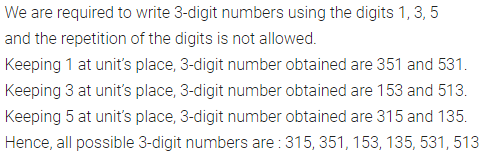Question 8.
Write all possible 3-digits numbers using the digits 7, 0 and 6. Repetition of digits is not allowed. Also, find their sum.
Solution: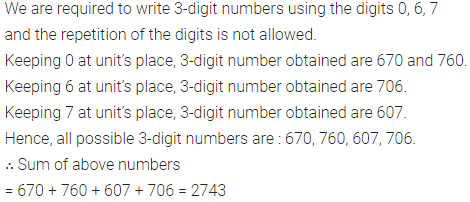Question 9.
Write all possible 2-digit numbers using the digits 4, 0 and 9. Repetition of digits is not allowed. Also, find their sum.
Solution:Question 10.
Write all possible 2-digit numbers that can be formed by using the digit 3, 7 and 9. Repetition of digits is allowed.
Solution:Question 11.
Write all possible numbers using the digits 3,1 and 5. Repetition of digits is not allowed.
Solution: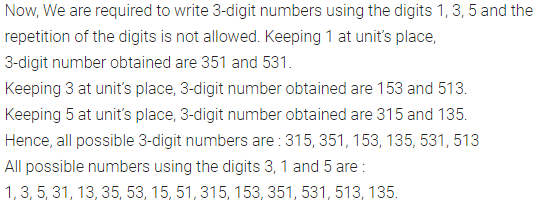Question 12.
How many 6-digit numbers are there in all ?
Solution: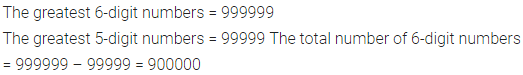Question 13.
Write down the greatest number and the smallest number of 4-digits that can be formed by the digits 7, 5,0 and 4 using each digit only once.
Solution: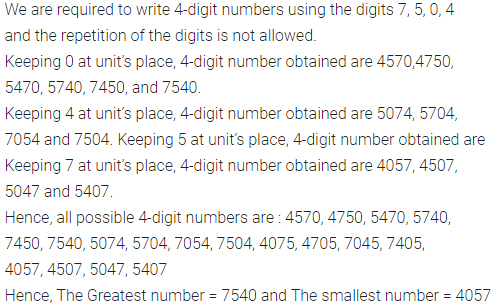Question 14.
Rearrange the digits of the number 5701024 to get the largest number and the smallest number of 7 digits.
Solution: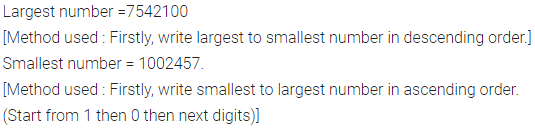Question 15.
Keeping the place value of digit 3 in the number 730265 same, rearrange
the digits of the given number to get the largest number and smallest number of 6 digits.
Solution:Question 16.
Form the smallest and greatest 4-digit numbers by using any one digit twice from the digits:
(i) 5, 2, 3, 9
(ii) 6, 0, 1, 4
(iii) 4, 6, 1, 5, 8.
Solution:Question 17.
Write
(i) the greatest number of 6 digits
(ii) the smallest number of 7 digits. Also find their difference.
Solution: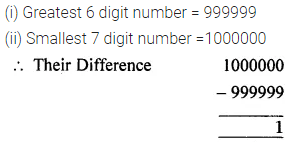Question 18.
Write the greatest 4-digit number of distinct digits.
Solution: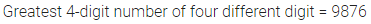Question 19.
Write the smallest 4-digit number of distinct digits.
Solution:Question 20.
Write the greatest 6-digit number using three different digits.
Solution: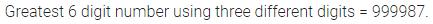Question 21.
Write the smallest 7-digit number using four different digits.
Solution: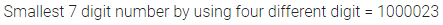Question 22.
Write the greatest and the smallest 4-digit numbers using four different digits with the conditions as given:
(i) Digit 7 is always at units place.
(ii) Digit 4 is always at tens place.
(iii) Digit 9 is always at hundreds place.
(iv) Digit 2 is always at thousands place.
Solution: